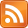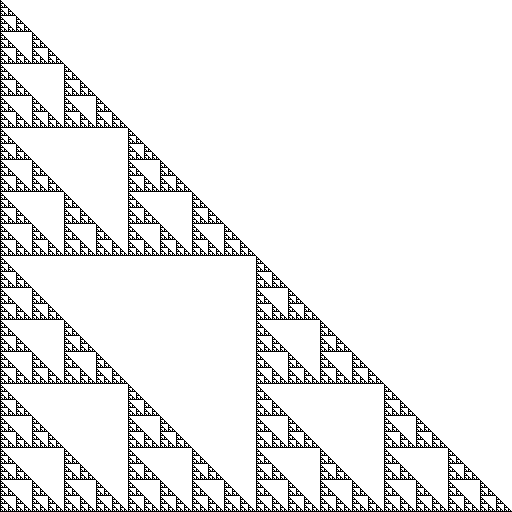Take the first five Fermat numbers (numbers of the form $2^{2^n} + 1$, $n \in \mathbb{N}$) and multiply them together in all possible combinations (without repetition). You will get $2^5 = 32$ results. Write them in binary, ordered smallest to largest, and you'll see that that pattern of zeroes and ones is the beginning rows of Sierpinski's Triangle.Wow! I learned this fact from a blog post and wanted to understand deeply why it was true. After I understood, I wanted to transmit my understanding in a way that wasn't just an explanation with words — so I made this demo. Some words follow anyway.

We start with the first two Fermat numbers, 3 ("11" in binary) and 5 ("101"), and generate new numbers according to the following rule: take the last Fermat number in the triangle, and multiply each previous row by it in the triangle, going from top to bottom. When you run out, introduce the next Fermat number (i.e. if your last number was $2^{2^n} + 1$, your next will be $2^{2^{n+1}} + 1$). Repeat.

This rule generates all possible combinations inductively; the previous rows are made of all possible combinations of the previous Fermat numbers, so when you add a new number to the mix, you just need to multiply each previous row by it in order to generate all possible combinations of the set including your new number. This makes sense because when you do this you multiply the number of rows by 2, and the number of possible combinations of n+1 objects is twice as many as the number of combinations of n objects. This rule also conveniently generates the numbers in order.

The Sierpinksi's Triangle structure arises because the Fermat numbers give enough "space" for the structure in the previous rows to repeat itself. What happens when we multiply a previous row $x$ by a Fermat number, which looks like $10...01$?

Split it apart: $x(10...01) = x(10...00) + x(1)$. We add some number of zeroes to the end of $x$, then add $x$ to the result. We've essentially replaced the leading $1$ of the Fermat number with a copy of $x$, while swapping out its last $\text{length}(x)$ digits with another copy of $x$. Copies of $x$ intrude from both sides, leading us to replicate the previous rows twice: one facing left, and one facing right. That's what gives rise to the fractal nature which is the hallmark of the triangle.

Note that this only works as long as there's enough space for the two copies not to interfere with one another; as long as we don't have to do any carries when we do the addition above. The Fermat numbers have an exponentially increasing number of zeroes between the 1s; if that number grew more slowly, there would be interference, since multiplying by each previous row each time means that we have an exponentially increasing number of rows to multiply by.